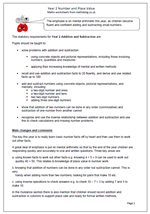# New Maths Curriculum: Year 2 Addition and SubtractionYear 2 Addition and subtraction

The emphasis is on mental arithmetic this year, as children become fluent and confident adding and subtracting small numbers.

The statutory requirements for Year 2 Addition and Subtraction are:

Pupils should be taught to:

•    solve problems with addition and subtraction:

using concrete objects and pictorial representations, including those involving numbers, quantities and measures

applying their increasing knowledge of mental and written methods

•    recall and use addition and subtraction facts to 20 fluently, and derive and use related facts up to 100

•    add and subtract numbers using concrete objects, pictorial representations, and mentally, including:
a two-digit number and ones
a two-digit number and tens
two two-digit numbers

•    show that addition of two numbers can be done in any order (commutative) and subtraction of one number from another cannot

•    recognise and use the inverse relationship between addition and subtraction and use this to check calculations and missing number problems.

The key this year is to really learn basic number facts off by heart and then use them to work out other facts.
A great deal of emphasis is put on mental arithmetic so that by the end of the year children are responding quickly and accurately to oral and written questions. Three key areas are:

a. using known facts to work out other facts e.g. knowing 4 + 5 = 9 can be used to work out
quickly 40 + 50. This relates to knowledge of place value in number work.

b. knowing that addition of numbers can be done in any order but subtraction cannot. This is very
handy when adding more than two numbers; looking for pairs that make 10 etc.

c. using inverse operations to check answers e.g. to check 10 – 7 = 3 by adding 7 and 3 to make 10.

In the Guidance section there is also mention that children should record addition and subtraction in columns to support place vale and ready for formal written methods.

New Maths Programme of Study: Year 2 Addition and Subtraction